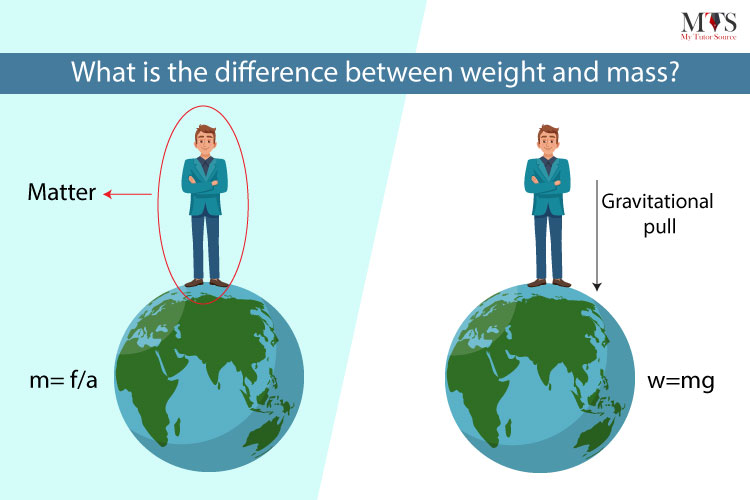# Differences between mass and weight

 S.N Mass Weight 1. The amount of matter contained in a body is called mass. The force by which earth attracts or pull objects towards its center is called weight. 2. It remains always constant regardless of the location in universe. It varies from place to place. 3. It is measured by ordinary or beam balance. It is measured by spring balance. 4. It is measured in grams or kilograms. It is measured in newton. 5. It has only magnitude but no direction so scalar quantity. It has both magnitude and direction so called vector. 6. Mass of a body can never be zero. Weight can be zero if gravity does not act on the body. 7. Mass of a moving body can be calculated by using formula: F=m/a. Weight of a body can be calculated by using  formula: w=m.g. 8. It is closely related to inertia. It is closely related to gravity. 9. It is denoted by ‘m’ or ‘M’. It is denoted by ‘w’.Image source: mytutorsource

References: